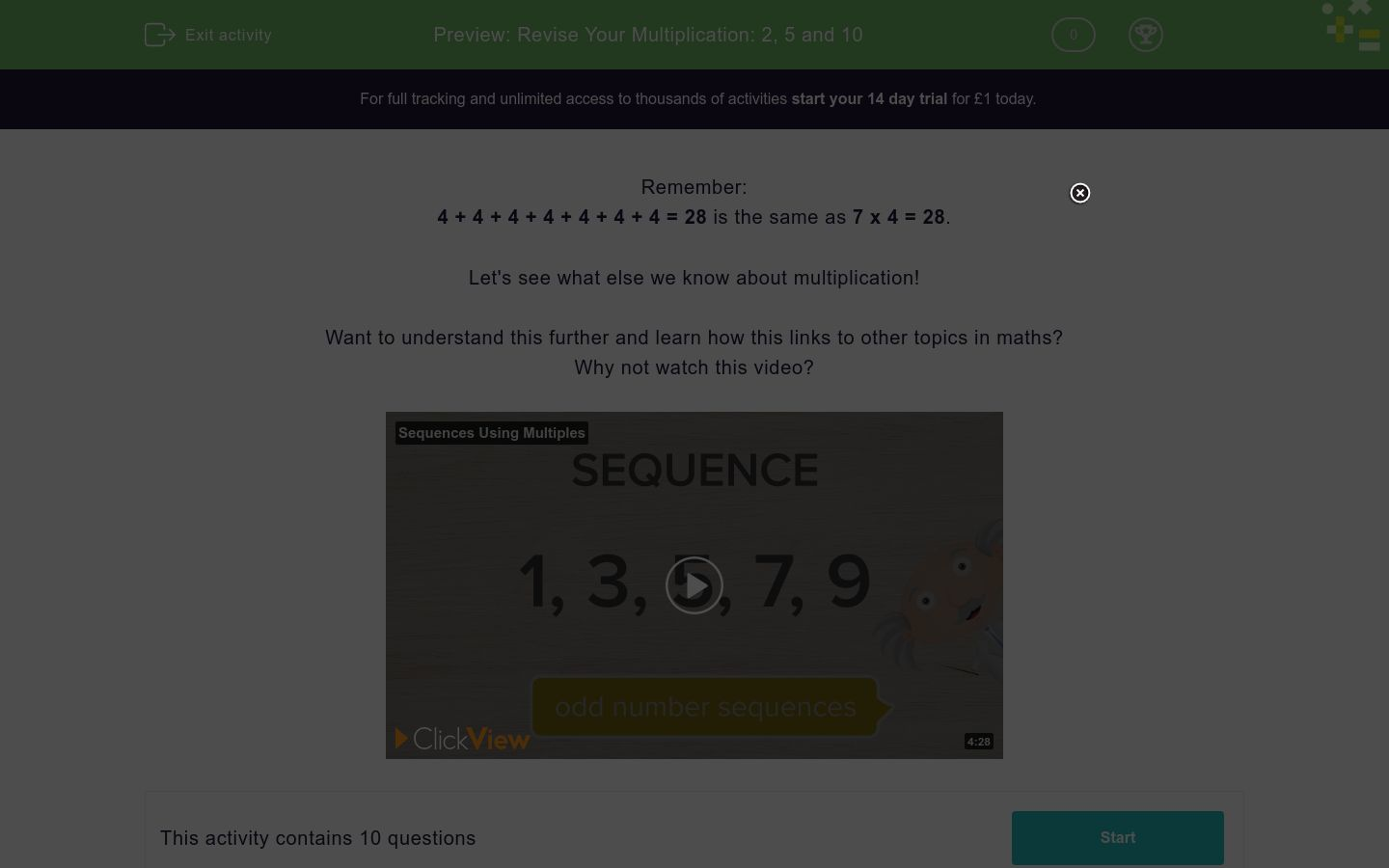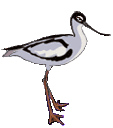# Revise Your Multiplication: 2, 5 and 10

In this worksheet, students revise multiplying by 2, 5 and 10.Key stage:  KS 1

Curriculum topic:   Number: Multiplication and Division

Curriculum subtopic:   Use Multiplication/Division Facts (2, 5, 10)

Difficulty level:### QUESTION 1 of 10

Remember:

4 + 4 + 4 + 4 + 4 + 4 + 4 = 28 is the same as 7 x 4 = 28.

Let's see what else we know about multiplication!

Want to understand this further and learn how this links to other topics in maths?
Why not watch this video?

What is 2 multiplied by 2?

Work out: 4 × 3

What is 7 times 2?

Work out: 6 × 10

Multiply 10 by 2

What is the missing number?

? × 10 = 80

What is the missing number?

? × 5 = 45

Is 2 × 10 the same as 10 × 2?

Yes

No

What is 5 times 10?Each bird has 2 legs.

What is 9 times 2? (this is the total number of legs)

• Question 1

What is 2 multiplied by 2?

4
EDDIE SAYS

2 x 2 = 4

• Question 2

Work out: 4 × 3

12
EDDIE SAYS

4 x 3 = 12

• Question 3

What is 7 times 2?

14
EDDIE SAYS

7 x 2 = 14

• Question 4

Work out: 6 × 10

60
EDDIE SAYS

6 x 10 = 60

• Question 5

Multiply 10 by 2

20
EDDIE SAYS

10 x 2 = 20

• Question 6

What is the missing number?

? × 10 = 80

8
EDDIE SAYS

80 ÷ 10 = 8

• Question 7

What is the missing number?

? × 5 = 45

9
EDDIE SAYS

45 ÷ 5 = 9

• Question 8

Is 2 × 10 the same as 10 × 2?

Yes
EDDIE SAYS

2 x 10 = 20

10 x 2 = 20
• Question 9

What is 5 times 10?

50
EDDIE SAYS

5 x 10 = 50

• Question 10Each bird has 2 legs.

What is 9 times 2? (this is the total number of legs)

18
EDDIE SAYS

9 × 2 = 18

2 + 2 + 2 + 2 + 2 + 2 + 2 + 2 + 2  = 18

---- OR ----

Sign up for a £1 trial so you can track and measure your child's progress on this activity.

### What is EdPlace?

We're your National Curriculum aligned online education content provider helping each child succeed in English, maths and science from year 1 to GCSE. With an EdPlace account you’ll be able to track and measure progress, helping each child achieve their best. We build confidence and attainment by personalising each child’s learning at a level that suits them.

Get started